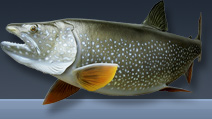# Using Co-ordinates

## Introduction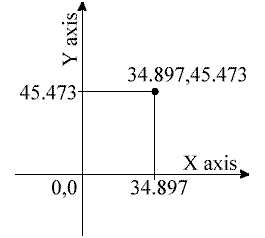A good understanding of how co-ordinates work in AutoCAD is absolutely crucial if you are to make the best use of the program. If you are not familiar with co-ordinates and co-ordinate systems, take some time to familiarise yourself with the basic concepts.

Co-ordinates fall into two types, namely Cartesian and Polar. A basic understanding of these co-ordinate types will help you to use AutoCAD to construct drawings more easily. In addition, these two co-ordinate types come in two distinct flavours. They can be either Absolute or Relative. Knowing just when and where to use the various types and flavours of co-ordinate is the key to efficient drawing with AutoCAD.

Top of page

## Cartesian Co-ordinates

Despite the fancy title (named after the French philosopher and mathematician René Descartes 1596-1650), the Cartesian co-ordinate system is the standard co-ordinate system. The position of a point can be described by its distance from two axes, X and Y. This results in a simple point description using two numbers separated by a comma e.g. 34.897,45.473.

In the example on the right the point described lies 34.897 drawing units to the right of the Y axis and 45.473 drawing units above the X axis. The first value (34.897) is known as the X co-ordinate because it's value is measured along the X axis. The second value is known as the Y co-ordinate because it's value is measured along the Y axis. The X and Y axes are two lines of infinite length which intersect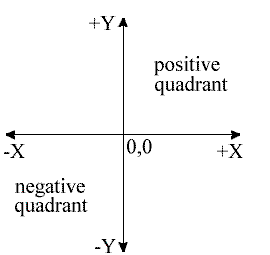at the origin point. The co-ordinate value of the origin point is always 0,0. When viewed in plan the X and Y axes are always perpendicular to one another with the X axis in a horizontal position and the Y axis in a vertical position (See illustration). X co-ordinate values become negative to the left of the Y axis and Y co-ordinate values become negative below the X axis. All co-ordinate values (both X and Y) are negative in the lower left hand quadrant and positive in the upper right hand quadrant. Normally we try to work in the positive quadrant. Although this is not essential for AutoCAD to operate, it does tend to make life easier because we don't need to worry about negative numbers.

AutoCAD allows you to use co-ordinates to draw objects rather than using pick points. For example you could draw a line like this:

#### Command Sequence

Command: LINE
From point: 34.897,45.473
To point: 54.896,65.395
To point:(to end)

This sequence draws a line between the two co-ordinate points specified. Note that if you enter a co-ordinate that is off the screen the line will still be drawn to the required point. You will then need to zoom out in order to see the whole line. You can also start the Line command by clicking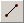on the Draw toolbar or from the pull-down menu ().

Top of page

## Polar Co-ordinates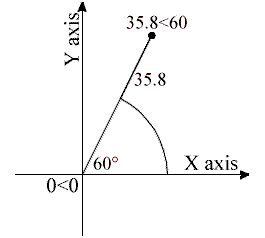Polar co-ordinates achieve the same result i.e. the description of the position of a point. The main difference is that polar co-ordinates use one distance and one angle to describe the position of a point rather than the two distances in the Cartesian system. The distance and angle measurements are made relative to an origin. This results in a point description which looks like this 34.897<30 where the first figure is the distance (in drawing units) and the second is the angle. Notice that the separator in the case of polar co-ordinates is the "less than" mathematical symbol. If you look at your keyboard you will see that this symbol is typed by using Shift and comma.

AutoCAD angles start at 3 o'clock (i.e. along the positive portion of the X axis) and increase in an anti-clockwise direction. You can specify negative angles if you need to define an angle in a clockwise direction although this is not really necessary because angles are circular, hence an angular value of -30 degrees will give the same result as an angular value of 330 degrees (there are 360 degrees in a full circle).

Top of page

## The UCS Icon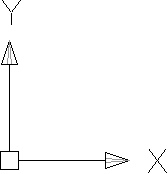In the bottom left hand corner of the AutoCAD drawing window you will see a symbol like the one shown on the right. This is called the UCS (User Co-ordinate System) icon and it is there to remind you which is the X axis and which is the Y axis. The empty box at the intersection of the X and Y axes is there to remind you that you are using "World" co-ordinates and that the UCS icon is not positioned over the true origin of the current co-ordinate system, probably because it is off screen. See the UCS Icon tutorial for more details on this feature.

Top of page

## Absolute & Relative Co-ordinates

Both Cartesian and polar co-ordinates come in two flavours, absolute and relative. The distinction is quite simple, absolute co-ordinates relate to the X and Y axes and the origin of the current co-ordinate system, whilst relative co-ordinates relate to the current pick point. When you are specifying co-ordinates you need to tell AutoCAD which type you want. Absolute co-ordinates are typed exactly as in the examples above. To specify a relative co-ordinate you need to use the "at" symbol as a prefix. In the case of the two examples above a relative Cartesian co-ordinate looks like this @34.897,45.473 and a relative polar co-ordinate looks like this @34.897<30. Relative co-ordinates are very useful for drawing objects which you know the size of. For example, you could draw a square of 12 units with its lower left hand point at 30,40 as follows:

#### Command Sequence

Command: LINE
From point: 30,40 (an absolute Cartesian co-ordinate)
To point: @0,12 (a relative Cartesian co-ordinate)
To point: @12<0 (a relative polar co-ordinate)
To point: @0,-12 (another relative Cartesian co-ordinate)
To point: C (to close)

Try this sequence out and watch the square drawn as you enter each co-ordinate value. You can also use this method to quickly draw a rectangle of known size. Say you needed to draw a rectangle 20 drawing units wide and 10 drawing units high and you didn't mind where exactly the rectangle is drawn, you could do this:

#### Command Sequence

Command: RECTANGLE
Specify first corner point or [Chamfer/Elevation/Fillet/Thickness/Width]: (pick a point near the middle of the drawing area)
Specify other corner point or [Dimensions]: @20,10

Note that the relative X co-ordinate determines the rectangle width and the relative Y co-ordinate determines the rectangle height.

See the Direct Distance Entry tutorial for more ways to draw lines of known length.

Top of page

## The Status BarThe status bar at the bottom of the AutoCAD window always shows the current co-ordinate value at the cursor point. Watch the co-ordinate values change as the cursor moves across the drawing area. You can turn the dynamic co-ordinate display off and on using the F6 key on the keyboard. See Function Keys on the Drawing Aids tutorial for more details.

Top of page